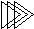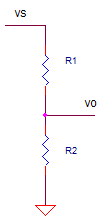Electronic Design with Excel
 Topics | VBA Basics | Back to eCircuit Center

RESISTOR DIVIDER

SIGNAL ATTENUATION

This is a simple example of using VBA for electronic design . You could ask - why  use a VBA function at all? But, even in this case, VBA makes analysis a little easier to explore. Here's the divider circuit.The gain (or, attenuation) of this circuit is given by

K = R2 / (R1 + R2)

Let's create a simple VBA function to perform this calculation (See VBA Basics).

 Function K_Rdiv1(R1, R2)   ' Gain of resistor divider   K_Rdiv1 = R2 / (R2 + R1)End Function

To see the VBA code hit ALT-F11 and double click on the Modules > Module1 in the VBA Project window. This opens the code window for this module.

DIVIDER ACCURACY

For this example, the divider is creating a precision +5V reference from an available +10V reference. The +5V level is used as a reference voltage for an ADC. But how accurate is this +5V level? Let's calculate the output given ideal components and then given initial tolerances of the +10V and resistors R1 and R2.

 Vs R1 R2 K Rdiv Vo Vo_err Error (%) 10.0 10000 10000 0.500 5.000 0.000 0.0 Ideal output 10.0 10100 10000 0.498 4.975 -0.025 -0.5 1% error in R1 10.0 10000 10100 0.502 5.025 0.025 0.5 1% error in R2 10.1 10000 10000 0.500 5.050 0.050 1.0 1% error in Vs

The cells in the K_Rdiv column hold the function call. For example, in the first entry in the K_Rdiv column looks like

=K_Rdiv1(C11,D11)

where C11 and D11 hold R1 and R2. Vo calculates the output as

vo = K_Rdiv * vs

And finally we calculate the error from the ideal result in both voltage and %.

The first row above, tells us the ideal story we want to believe. With ideal resistors R1=R2=10k you get a gain of 0.500 and vo = 5.000V. But what happens if you place real resistors with an initial 1% tolerance in the circuit? The entry for a 1% error for R1 was typed in the second row as 10000*1.01. What kind of error did it produce? This resulted in a 0.5% error. You can also see the influence of errors for R2 and vs. Interesting how the resistors error effects the output by half their tolerance. On the other hand the voltage va sources error effects the output directly by its tolerance.

The best part of VBA is that it lets you play with the code. Once you see some code, jump right in and start messing with it or write a new function altogether. Just copy the existing code to a space just below the original. Then start modifying the name and calculations. Here are a few ideas you can try:

1. Write a function to calculate the parallel combo of two resistors. You can name the function something like "Function R_Par(R1, R2)".
2. Make a function to calculate the non-inverting gain of an op amp.